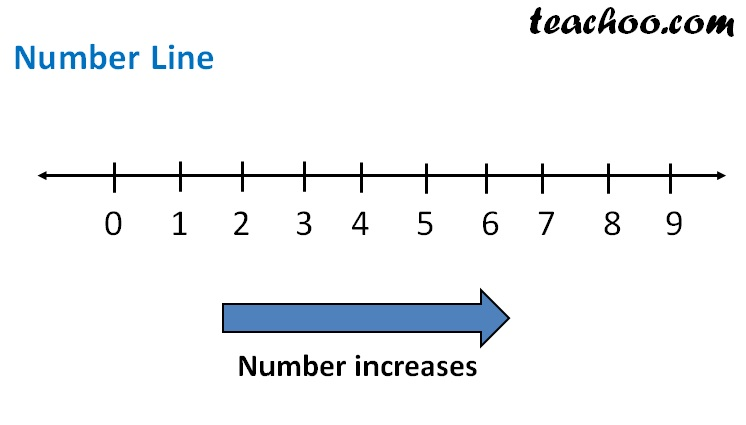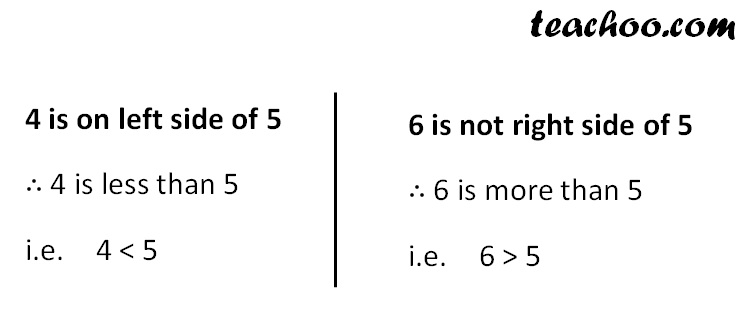Whole numbers on number line

Chapter 2 Class 6 Whole Numbers
Concept wiseNote

Smaller number is on left side

Larger Number is on Right SideLearn in your speed, with individual attention - Teachoo Maths 1-on-1 Class

### Transcript

Note Smaller number is on left side Larger Number is on Right Side 4 is on left side of 5 ∴ 4 is less than 5 i.e. 4 < 5 6 is not right side of 5 ∴ 6 is more than 5 i.e. 6 > 5 So, < means Less than > means More than Whole numbers between two numbers How many whole numbers are there between 4 & 7? Method 1 4, 5, 6, 7 So, there are 2 numbers between 4 & 7 Method 2 Numbers between 4 & 7 = (7 – 4) – 1 = 3 – 1 = 2 So, there are 2 numbers between 4 & 7 Method 2 Numbers between 4 & 7 = (7 – 4) – 1 = 3 – 1 = 2 So, there are 2 numbers between 4 & 7